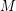# Fixed-point logic with counting

 Importance: Medium ✭✭
 Author(s): Blass, Andreas
 Subject: Logic » Finite Model Theory
 Keywords: Capturing PTime counting quantifiers Fixed-point logic FMT03-Bedlewo
\item Given a graph, does it have a perfect matching, i.e., a setof edges such that every vertex is incident to exactly one edge from? \item Given a square matrix over a finite field (regarded as a structure in the natural way, as described in [BGS02]), what is its determinant?